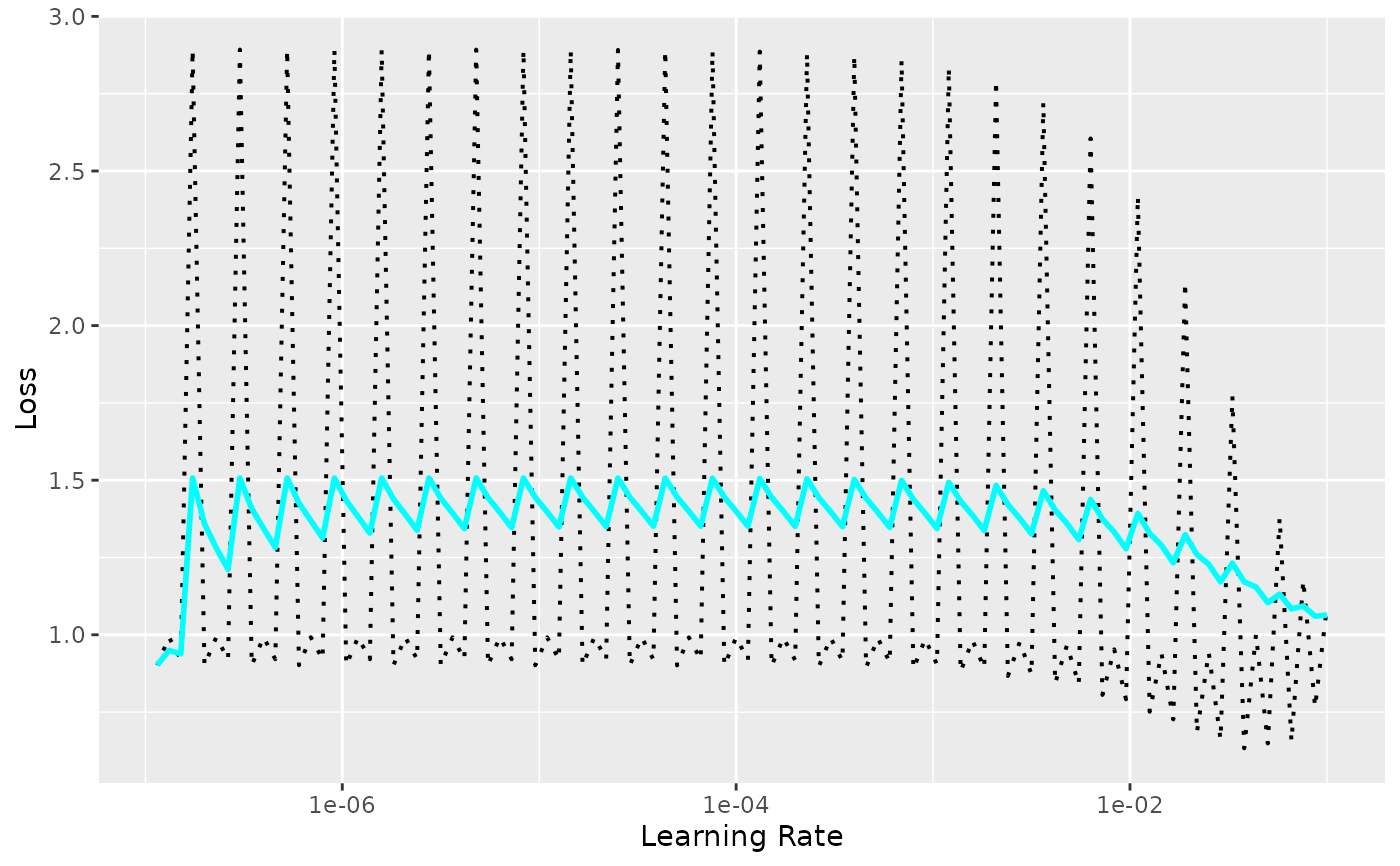Learning Rate Finder

## Usage

lr_finder(
object,
data,
steps = 100,
start_lr = 1e-07,
end_lr = 0.1,
log_spaced_intervals = TRUE,
...,
verbose = NULL
)

## Arguments

object

An nn_module that has been setup().

data

steps

(integer) The number of steps to iterate over in the learning rate finder. Default: 100.

start_lr

(float) The smallest learning rate. Default: 1e-7.

end_lr

(float) The highest learning rate. Default: 1e-1.

log_spaced_intervals

(logical) Whether to divide the range between start_lr and end_lr into log-spaced intervals (alternative: uniform intervals). Default: TRUE

...

Other arguments passed to fit.

verbose

Wether to show a progress bar during the process.

## Value

A dataframe with two columns: learning rate and loss

## Examples

if (torch::torch_is_installed()) {
library(torch)
ds <- torch::tensor_dataset(x = torch_randn(100, 10), y = torch_randn(100, 1))
dl <- torch::dataloader(ds, batch_size = 32)
model <- torch::nn_linear
model <- model %>% setup(
loss = torch::nn_mse_loss(),